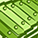# QlikView App Dev

Discussion Board for collaboration related to QlikView App Development.

Announcements
Join this live chat April 6, 10AM EST - QlikView to Qlik Sense REGISTER
cancel
Showing results for
Search instead for
Did you mean:Creator II

## %change calculation

Hi Experts,

Can any one please help me on calculation of %change.

In the excel the %change is calculated from Frequency aggregated by month as

N/A

=(I10-I9)*100/I9

=(I11-I10)*100/I10

=(I12-I11)*100/I11

Please help me on getting this calculation into Qlik.

1 Solution

Accepted SolutionsPartner

you can use either previous / peek functions in the script or the Above function on the chart

in script you sort your data by date then in a successive reload you wirte:

if (rowNO() = 1,null(),100*(Frequency - previous(Frequency)) / previous(Frequency)) as %Change

on the chart you would write: 100 * (Sum(Frequency) - Above(Sum(Frequency))) / above(Sum(Frequency))

or Sum(Frequency) / Above(Sum(Frequency)) - 1

and you set formatting to display as percentage in the NUMBER tab of the chart's properties

I can walk on water when it freezes
2 RepliesPartner

you can use either previous / peek functions in the script or the Above function on the chart

in script you sort your data by date then in a successive reload you wirte:

if (rowNO() = 1,null(),100*(Frequency - previous(Frequency)) / previous(Frequency)) as %Change

on the chart you would write: 100 * (Sum(Frequency) - Above(Sum(Frequency))) / above(Sum(Frequency))

or Sum(Frequency) / Above(Sum(Frequency)) - 1

and you set formatting to display as percentage in the NUMBER tab of the chart's properties

I can walk on water when it freezesMVP

Should be like this

Sum(Frequency)/Above(Sum(Frequency)) - 1